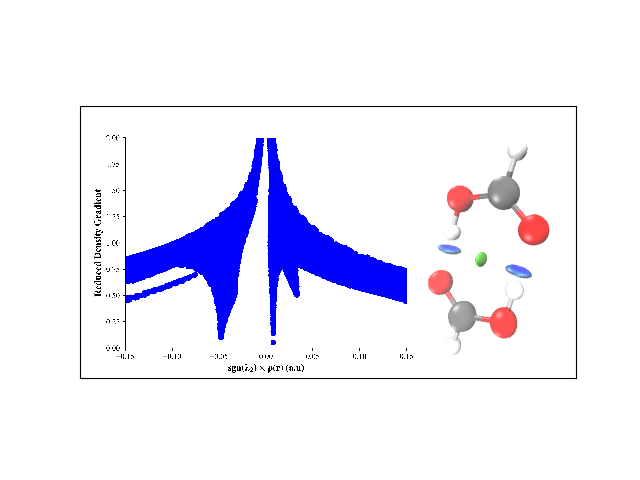# EX2: NCI from wave-function file and user-defined cube¶

Compute NCI and visualize it for formic acid dimer.from chemtools import NCI, UniformGrid

# 1. Build UniformGrid and NCI model

cub = UniformGrid.from_file('formic_acid_dimer.fchk', spacing=0.1, extension=2.0)
nci = NCI.from_file('formic_acid_dimer.fchk', grid=cub)

# 2. Generate plot, cube file(s) and script for visualizing NCI
#    Files generated are formic_acid_dimer-dens.cube, formic_acid_dimer-grad.cube,
#    & formic_acid_dimer.vmd
#    To visualize the iso-surface, use command: \$ vmd -e formic_acid_dimer.vmd

# nci.generate_plot('formic_acid_dimer', denslim=(-0.15, 0.15))
nci.generate_scripts('formic_acid_dimer')


Total running time of the script: ( 1 minutes 14.558 seconds)

Generated by Sphinx-Gallery